﻿ 随机激励作用下电力系统机电振荡机理解析 Analysis of the Mechanism of Electromechanical Oscillations for Power System under Random Excitation

Journal of Electrical Engineering
Vol. 07  No. 02 ( 2019 ), Article ID: 30920 , 8 pages
10.12677/JEE.2019.72017

Analysis of the Mechanism of Electromechanical Oscillations for Power System under Random Excitation

Jintao Jiang

School of Electrical Engineering, Northeast Dianli University, Jilin Jilin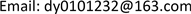Received: June 2nd, 2019; accepted: June 17th, 2019; published: June 24th, 2019ABSTRACT

There is a wealth of dynamic information hidden in system responses which are subject to the random excitations. On the basis of the stochastic differential algebraic equations and features of random excitations, by linearizing the equations, this paper derivates the analytical expressions of the power system dynamic response under random excitation. So we mathematically prove the presence of power oscillation characteristics in power ambient data. It reveals the basic mechanism of system oscillation characteristics by using ambient signals. Based on the case of small fluctuations caused by random changes of loads of IEEE-four generators two areas system, comparing the identified parameters with theoretical characteristic parameters and analysis of the results of probability distributions, we conclude that it is feasible and effective to identify the oscillation characteristics by using the ambient data. Our work further reinforces the theoretical basis of the oscillation characteristics identification on the basis of the ambient signal.

Keywords:Random Excitation, Low Frequency Oscillation, Ambient Signal, Mode ParametersCopyright © 2019 by author and Hans Publishers Inc.1. 引言

2. 电力系统动态响应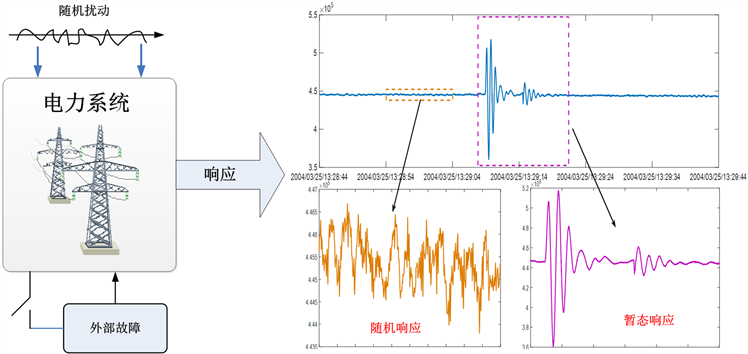Figure 1. Power system response type

3. 随机激励下电力系统响应的数学解析

$\left\{\begin{array}{l}\stackrel{˙}{x}=f\left(x,y\right)\text{\hspace{0.17em}}\text{\hspace{0.17em}}\text{\hspace{0.17em}}\text{\hspace{0.17em}}\text{\hspace{0.17em}}\text{\hspace{0.17em}}\text{\hspace{0.17em}}\text{\hspace{0.17em}}\text{\hspace{0.17em}}\text{\hspace{0.17em}}\text{\hspace{0.17em}}\text{\hspace{0.17em}}\text{\hspace{0.17em}}\text{\hspace{0.17em}}\text{\hspace{0.17em}}\text{\hspace{0.17em}}\text{\hspace{0.17em}}\text{\hspace{0.17em}}\text{\hspace{0.17em}}\text{\hspace{0.17em}}\text{\hspace{0.17em}}\text{\hspace{0.17em}}\text{\hspace{0.17em}}\text{\hspace{0.17em}}\text{\hspace{0.17em}}\text{\hspace{0.17em}}\text{\hspace{0.17em}}\text{\hspace{0.17em}}\text{\hspace{0.17em}}\text{\hspace{0.17em}}\text{\hspace{0.17em}}\text{\hspace{0.17em}}\text{\hspace{0.17em}}\text{\hspace{0.17em}}\text{\hspace{0.17em}}\text{\hspace{0.17em}}\text{\hspace{0.17em}}\text{\hspace{0.17em}}\text{\hspace{0.17em}}\text{\hspace{0.17em}}\text{\hspace{0.17em}}\text{\hspace{0.17em}}\text{\hspace{0.17em}}\text{\hspace{0.17em}}\text{\hspace{0.17em}}\text{\hspace{0.17em}}\text{\hspace{0.17em}}\text{\hspace{0.17em}}\text{\hspace{0.17em}}\text{\hspace{0.17em}}\text{\hspace{0.17em}}\text{\hspace{0.17em}}\text{\hspace{0.17em}}\text{\hspace{0.17em}}\text{\hspace{0.17em}}\text{\hspace{0.17em}}\text{\hspace{0.17em}}\text{\hspace{0.17em}}\text{\hspace{0.17em}}\text{\hspace{0.17em}}\text{\hspace{0.17em}}\text{\hspace{0.17em}}\text{\hspace{0.17em}}\text{\hspace{0.17em}}\text{\hspace{0.17em}}\text{\hspace{0.17em}}\text{\hspace{0.17em}}\text{\hspace{0.17em}}\text{\hspace{0.17em}}\text{\hspace{0.17em}}\text{\hspace{0.17em}}\left(1\right)\\ 0=g\left(x,y\right)\text{\hspace{0.17em}}\text{\hspace{0.17em}}\text{\hspace{0.17em}}\text{\hspace{0.17em}}\text{\hspace{0.17em}}\text{\hspace{0.17em}}\text{\hspace{0.17em}}\text{\hspace{0.17em}}\text{\hspace{0.17em}}\text{\hspace{0.17em}}\text{\hspace{0.17em}}\text{\hspace{0.17em}}\text{\hspace{0.17em}}\text{\hspace{0.17em}}\text{\hspace{0.17em}}\text{\hspace{0.17em}}\text{\hspace{0.17em}}\text{\hspace{0.17em}}\text{\hspace{0.17em}}\text{\hspace{0.17em}}\text{\hspace{0.17em}}\text{\hspace{0.17em}}\text{\hspace{0.17em}}\text{\hspace{0.17em}}\text{\hspace{0.17em}}\text{\hspace{0.17em}}\text{\hspace{0.17em}}\text{\hspace{0.17em}}\text{\hspace{0.17em}}\text{\hspace{0.17em}}\text{\hspace{0.17em}}\text{\hspace{0.17em}}\text{\hspace{0.17em}}\text{\hspace{0.17em}}\text{\hspace{0.17em}}\text{\hspace{0.17em}}\text{\hspace{0.17em}}\text{\hspace{0.17em}}\text{\hspace{0.17em}}\text{\hspace{0.17em}}\text{\hspace{0.17em}}\text{\hspace{0.17em}}\text{\hspace{0.17em}}\text{\hspace{0.17em}}\text{\hspace{0.17em}}\text{\hspace{0.17em}}\text{\hspace{0.17em}}\text{\hspace{0.17em}}\text{\hspace{0.17em}}\text{\hspace{0.17em}}\text{\hspace{0.17em}}\text{\hspace{0.17em}}\text{\hspace{0.17em}}\text{\hspace{0.17em}}\text{\hspace{0.17em}}\text{\hspace{0.17em}}\text{\hspace{0.17em}}\text{\hspace{0.17em}}\text{\hspace{0.17em}}\text{\hspace{0.17em}}\text{\hspace{0.17em}}\text{\hspace{0.17em}}\text{\hspace{0.17em}}\text{\hspace{0.17em}}\text{\hspace{0.17em}}\text{\hspace{0.17em}}\text{ }\text{\hspace{0.17em}}\text{\hspace{0.17em}}\text{\hspace{0.17em}}\text{\hspace{0.17em}}\text{\hspace{0.17em}}\left(2\right)\end{array}$

$\stackrel{˙}{u}=-Cu+\delta \xi$ (5)

$\Delta y=\left[\begin{array}{cc}-{g}_{y}^{-1}{g}_{x}& -{g}_{y}^{-1}{g}_{u}\end{array}\right]\left[\begin{array}{l}\Delta x\\ \Delta u\end{array}\right]$ (6)

$\left[\begin{array}{l}\Delta \stackrel{˙}{x}\\ \Delta \stackrel{˙}{u}\end{array}\right]=\left[\begin{array}{l}{f}_{x}-{f}_{y}{g}_{y}^{-1}{g}_{x}-{f}_{y}{g}_{y}^{-1}{g}_{u}\\ 0-C\end{array}\right]\left[\begin{array}{l}\Delta x\\ \Delta u\end{array}\right]+\delta \left[\begin{array}{l}0\\ {I}_{{n}_{u}}\end{array}\right]\xi$ (7)

$\stackrel{˙}{z}=Az+\delta B\xi$ (8)

$|A-\lambda I|=0$ ，可计算出系统n个振荡模式对应的特征值 ${\lambda }_{i}={\sigma }_{i}+j{\omega }_{i}\left(i=1,2,\cdots ,n\right)$ ，则系统状态变量的时域解析表达式为：

$z\left(t\right)=\underset{i=1}{\overset{n}{\sum }}{v}_{i}{u}_{i}^{\text{T}}z\left(0\right){\text{e}}^{{\sigma }_{i}t}\mathrm{sin}\left({\omega }_{i}t+{\phi }_{i}\right)+\delta B\xi$ (9)

4. 实验结果与分析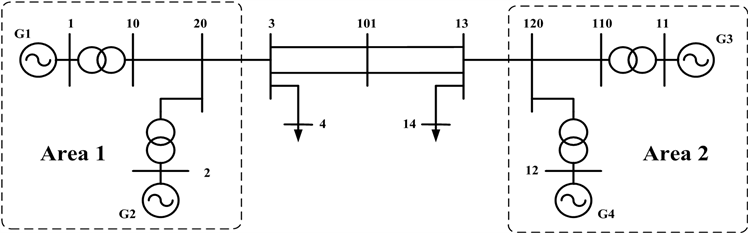Figure 2. The one-line diagram of four generators two areaTable 1. The characteristic results of four generators two area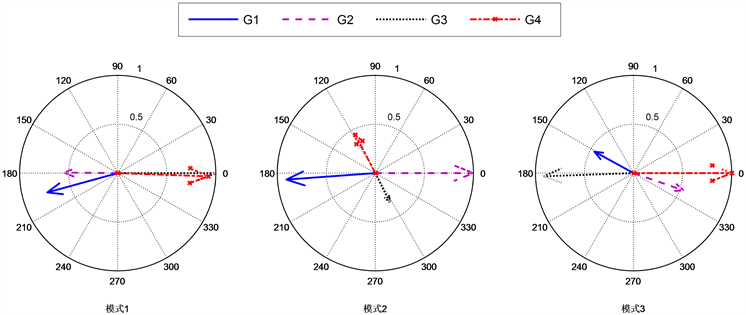Figure 3. Modal graph of four generators two area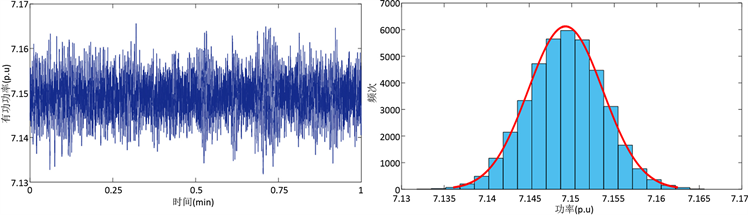Figure 4. The response and probability distribution of active power of generator 1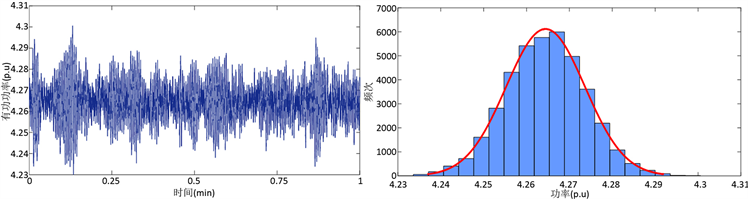Figure 5. The response and probability distribution of active power of inter area connected lineTable 2. The statistical results of active power of four generators and connected lineTable 3. The identification results of four generators two area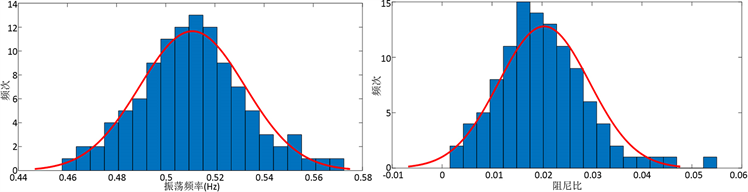Figure 6. The probability distributions of identification results of interarea mode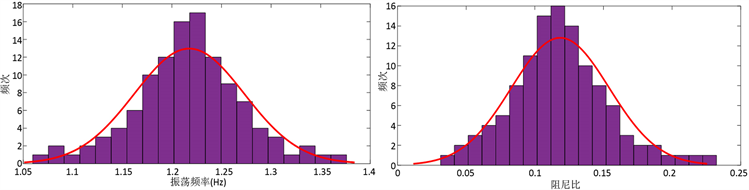Figure 7. The probability distributions of identification results of local mode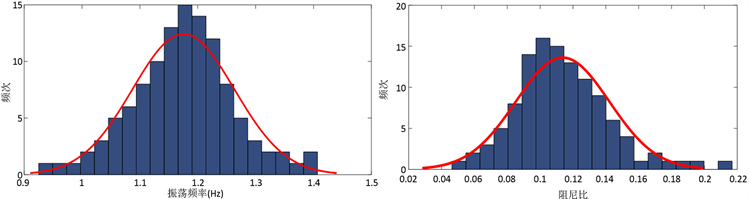Figure 8. The probability distributions of identification results of local mode

5. 结论

1) 随机激励下系统基本运行方式维持不变，在无新设备投运的前提下系统保持原有动态特征；

2) 采用合适的辨识技术能够从随机相应数据中提取出系统机电振荡参数，所提取参数及各机电振荡模态表征与基础运行方式吻合；

3) 实测数据分析结果表明基于随机响应数据的机电振荡特征识别对电力系统小干扰稳定在线量化评估具有重要的指导意义；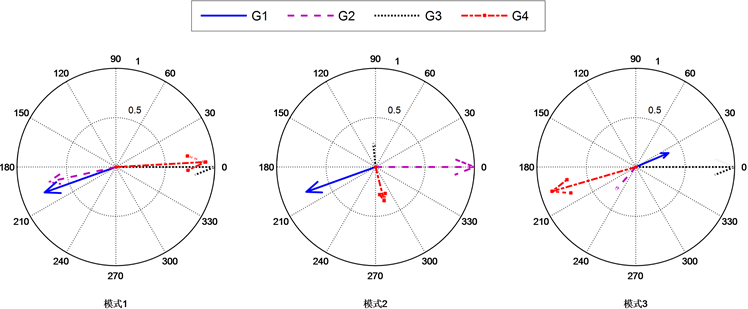Figure 9. Mode shape identification results of four generators two area

Analysis of the Mechanism of Electromechanical Oscillations for Power System under Random Excitation[J]. 电气工程, 2019, 07(02): 136-143. https://doi.org/10.12677/JEE.2019.72017

1. 1. Kundar, P., Paserba, J., Ajjarapu, V., et al. (2004) Definition and Classification of Power System Stability. IEEE Transactions on Power Systems, 19, 1387-1401. https://doi.org/10.1109/TPWRS.2004.825981

2. 2. 余贻鑫, 李鹏. 大区电网弱互联对互联系统阻尼和动态稳定性的影响[J]. 中国电机工程学报, 2005, 25(11): 6-11.

3. 3. 蔡国伟, 王丽馨, 杨德友. 环境激励下基于频域特征的扰动源定位方法[J]. 电力自动化设备, 2018, 38(6): 144-151.

4. 4. Kunder, P. (1944) Power System Stability and Control. McGraw-Hill, New York.

5. 5. Pierre, J., Trudnowski, D. and Canizares, C. (2012) Identification of Electromechanical Modes in Power Systems. IEEE Task Force Report.

6. 6. 杨德友, 蔡国伟, 陈家荣. 基于动态响应的机电振荡特征识别与能控性评估方法[J]. 电力自动化设备, 2014, 34(2): 54-60.

7. 7. 杨德友, 王文嘉, 高际惟, 王丽馨, 蔡国伟. 随机数据驱动下的机电振荡参数在线提取与阻尼调制(一): 基于ORSSI的模态参数在线辨识[J]. 中国电机工程学报, 2018, 38(8): 2253-2261+2535.

8. 8. Praveen, T., Srivastava, S.C. and Singh, S.N. (2011) A Modified TLS-ESPRIT-Based Method for Low-Frequency Mode Identification in Power Systems Utilizing Synchrophasor Measurements. IEEE Transactions on Power Systems, 26, 719-727. https://doi.org/10.1109/TPWRS.2010.2055901

9. 9. Nezam Sarmadi, S.A. and Vaithoanathan, V. (2014) Electromechanical Mode Estimation Using Recursive Adaptive Stochastic Subspace Identification. IEEE Transactions on Power Systems, 29, 349-358. https://doi.org/10.1109/TPWRS.2013.2281004

10. 10. Liu, G. and Venkatasubramanian, V.M. (2008) Oscillation Monitoring from Ambient PMU Measurements by Frequency Domain Decomposition. IEEE International Symposium on Circuits and Systems, Seattle, 18-21 May 2008, Vol. 27, 2821-2824.

11. 11. Zhou, N., Pierre, J.W. and Hauer, J.F. (2006) Initial Results in Power System Identification from Injected Probing Signals Using a Subspace Method. IEEE Transactions on Power Systems, 21, 1296-1302. https://doi.org/10.1109/TPWRS.2006.879292

12. 12. Bernt, K. (2003) Stochastic Differential Equations. 6th Edition, Springer Press, New York.

13. 13. Fedetico, M. and Rafacel, Z. (2013) A Systematic Method to Model Power Systems as Stochastic Differential Algebraic Equations. IEEE Transactions on Power Systems, 28, 4537-4544. https://doi.org/10.1109/TPWRS.2013.2266441